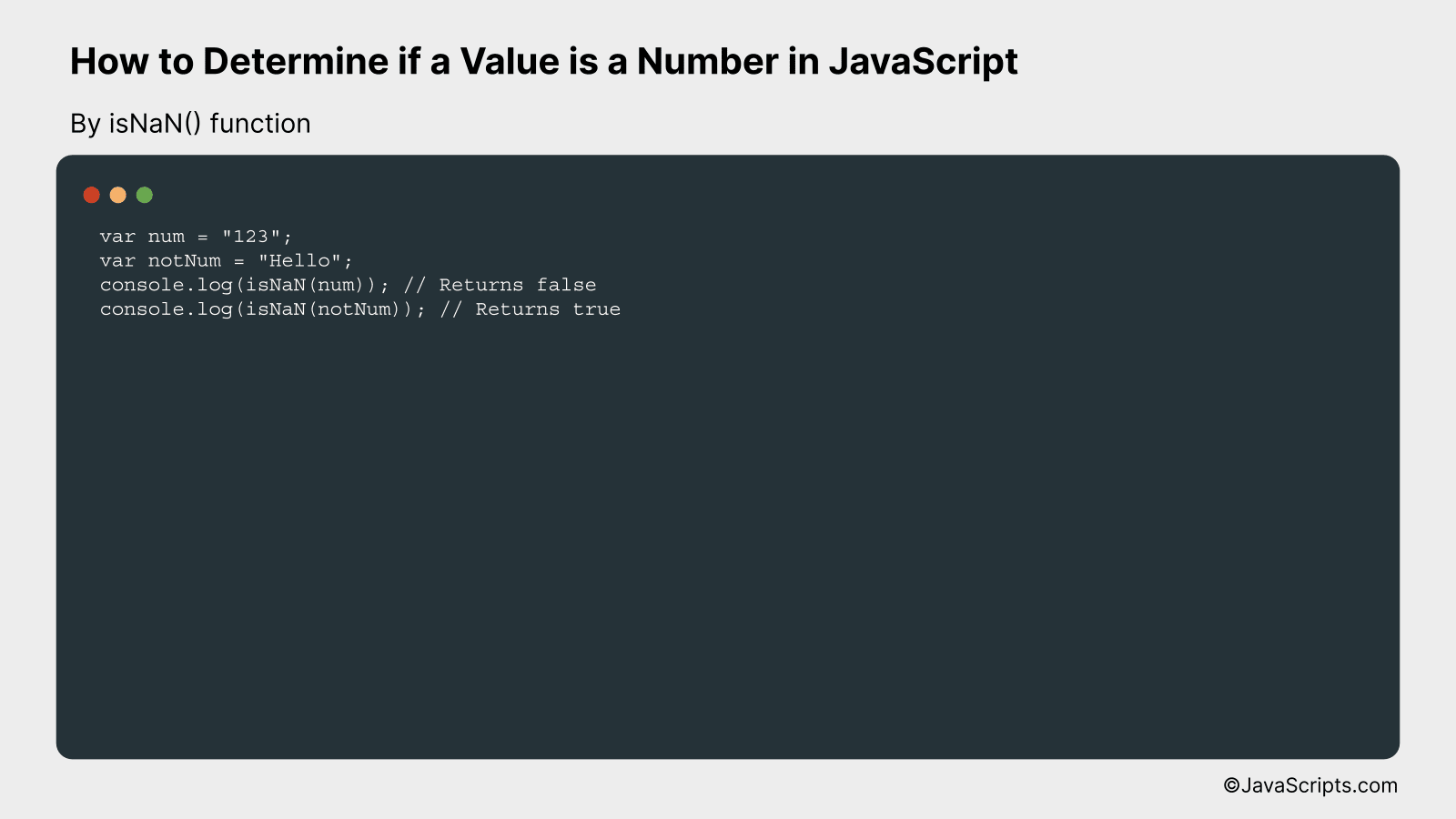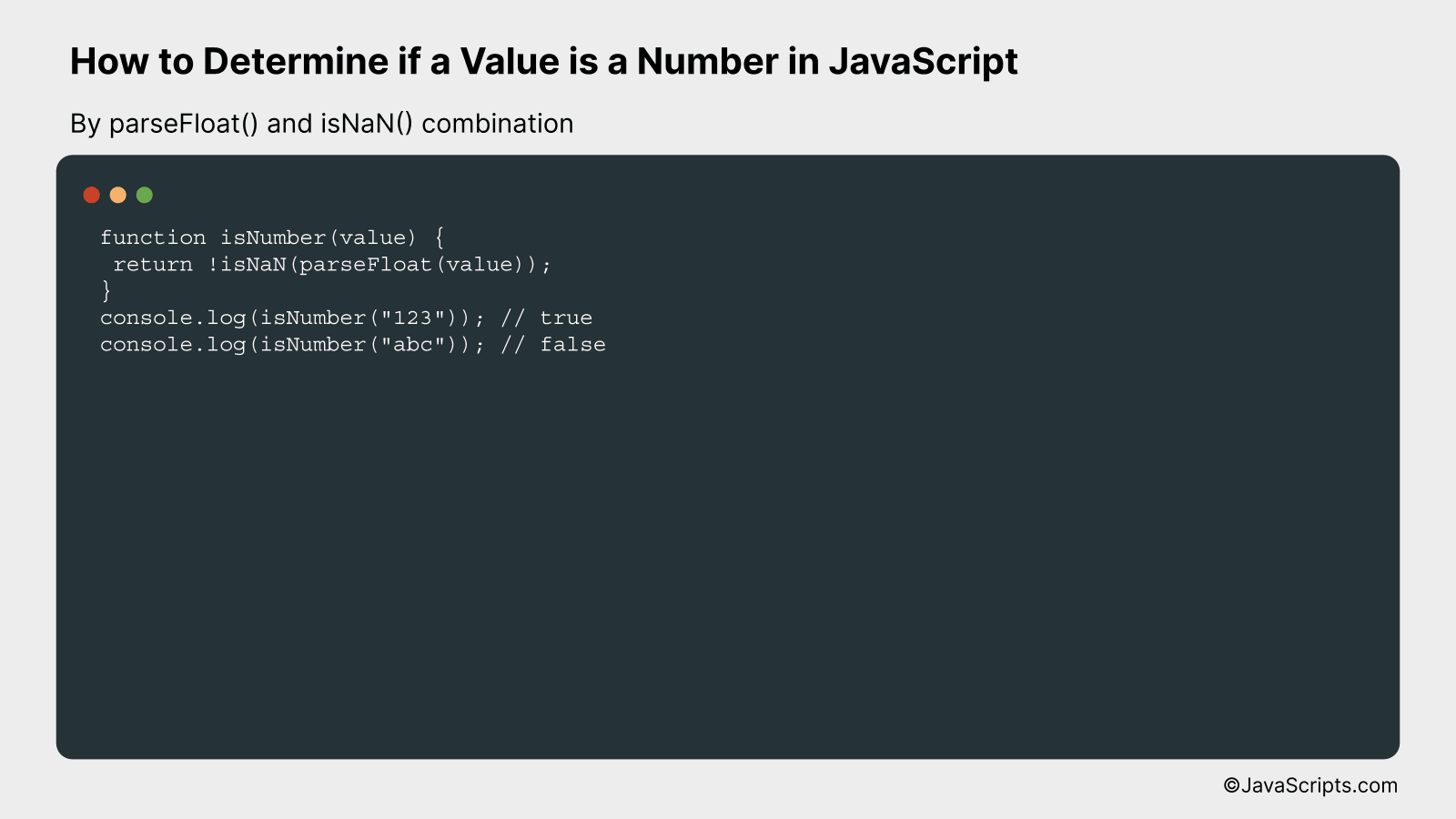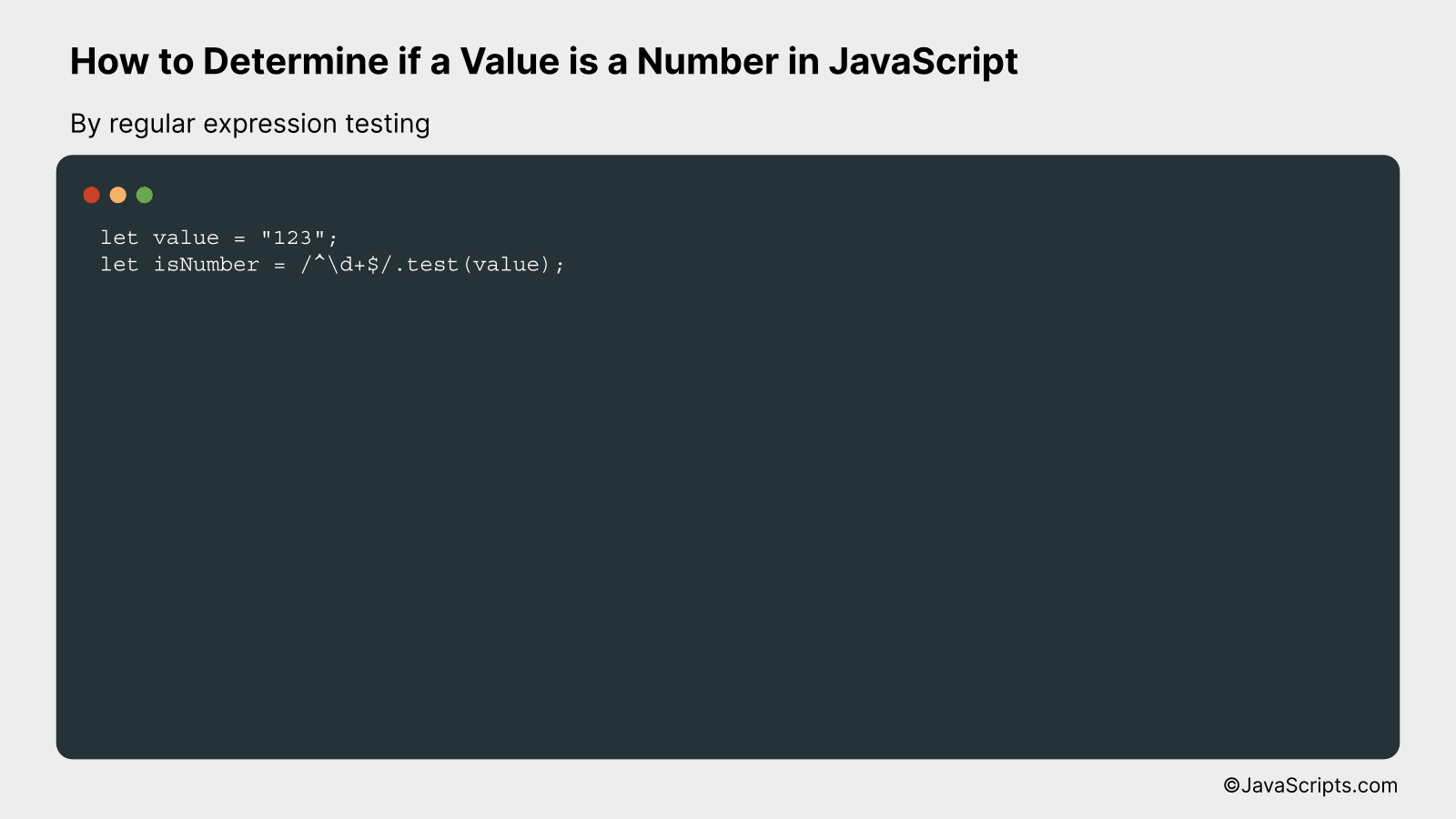# How to Determine if a Value is a Number in JavaScript

When working with JavaScript, you may find yourself needing to distinguish between numbers and other data types. Perhaps you’re validating user input or handling data received from an API or other source. Whatever the case may be, there’s a need for clarity and certainty; we have to be sure that a particular value is indeed a number.

So, how do you, as a programmer, ascertain this? How do you tell if a certain value is a real number and not just a string that looks like one? Let’s break it down together, making it as simple and as clear as possible to help you understand and apply in your coding journey.

In this post, we will be looking at the following 3 ways to determine if a value is a number in JavaScript:

• By isNaN() function
• By parseFloat() and isNaN() combination
• By regular expression testing

Let’s explore each…

## #1 – By isNaN() functionThe isNaN() function in JavaScript checks if a value is not a number. It returns true if the value is NaN (not a number), and false if the value is a number. For example, isNaN(123) will return false, whereas isNaN(‘Hello’) will return true.

``````
var num = "123";
var notNum = "Hello";
console.log(isNaN(num)); // Returns false
console.log(isNaN(notNum)); // Returns true
``````

### How it works

The isNaN() function works by attempting to convert the input into a number. If the conversion results in NaN (Not a Number), it returns true, indicating that the input is not a number. If the conversion is successful, it returns false, indicating that the input is a number.

• First, two variables `num` and `notNum` are defined. The `num` variable is assigned the string “123”, and the `notNum` variable is assigned the string “Hello”.
• Then, the isNaN() function is called with `num` as the argument. The function tries to convert “123” into a number, which is successful, so it returns false. This is logged to the console.
• Next, the isNaN() function is called with `notNum` as the argument. The function tries to convert “Hello” into a number, which is not possible, so it returns true. This is logged to the console.
• Therefore, by using the isNaN() function, we can determine whether a value is a number or not in JavaScript.

## #2 – By parseFloat() and isNaN() combinationWe can determine if a value is a number in JavaScript by using the parseFloat() and isNaN() functions. Firstly, the value is converted into a floating-point number with parseFloat(). Then, isNaN() is used to check if the converted value is a number or not. If isNaN() returns false, it indicates that the initial value was a number. Here is an example:

``````
function isNumber(value) {
return !isNaN(parseFloat(value));
}
console.log(isNumber("123")); // true
console.log(isNumber("abc")); // false
``````

### How it works

The provided value is passed to the parseFloat() function, which tries to convert this value into a floating-point number. If it can be converted, it returns the number; otherwise, it returns NaN (Not a Number). This returned value is then checked by the isNaN() function, which returns true if the argument is NaN and false if the argument is a number. In our function, we use the logical NOT operator (!) to reverse this boolean value, so that true is returned if the argument is a number and false if it’s not.

• Step 1: The function receives a value as an argument.
• Step 2: The parseFloat() function attempts to convert this value into a floating-point number.
• Step 3: The isNaN() function checks if the result of the parseFloat() function is Not a Number (NaN).
• Step 4: If the value is NaN, isNaN() will return true. However, the logical NOT operator (!) reverses this, hence it results in false. This means the initial value was not a number.
• Step 5: If the value is a number, isNaN() will return false. The logical NOT operator (!) reverses this, hence it results in true. This means the initial value was a number.

## #3 – By regular expression testingWe can check if a value is a number in JavaScript by using a regular expression that matches number patterns, and then test this against our value. For instance, if we want to check if “123” is a number, we’d use this approach.

``````
let value = "123";
let isNumber = /^d+\$/.test(value);
```
```

### How it works

The regular expression `/^d+\$/` checks if a string only contains digits (0-9). The `test` method returns a boolean indicating whether or not the string passes the regular expression’s condition.

• `/^d+\$/`: This is the regular expression we’re testing our string against. The `^` symbol means the start of the string, `d+` denotes one or more digits, and `\$` symbolizes the end of the string. So the entire expression checks if the string starts, continues and ends with one or more digits, meaning it’s entirely made up of numbers.
• `value`: This is the string we’re checking to see if it’s purely numeric.
• `test`: This is a method that tests for a match in a string. It returns `true` if it finds a match, else it returns `false`.
• `isNumber`: This will be a boolean value. If the value is entirely numeric, `isNumber` will be `true`, else it will be `false`.

Related:

In conclusion, figuring out whether a value is a number in JavaScript is not an uphill task. With methods like ‘isNaN’, ‘Number.isFinite’, and ‘typeof’, you can easily and effectively achieve this.

Remember, the key is to understand which method to use based on your specific requirement or scenario. By mastering these JavaScript methods, you can seamlessly handle numerical values and avoid errors in your codes.